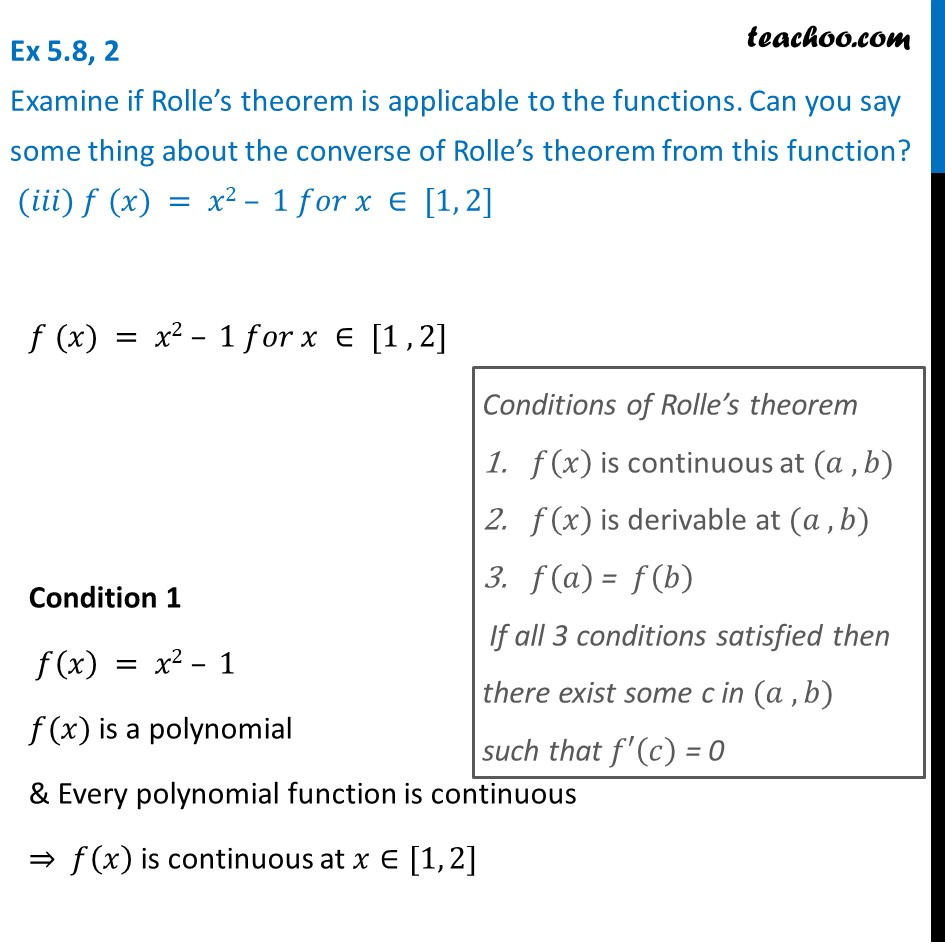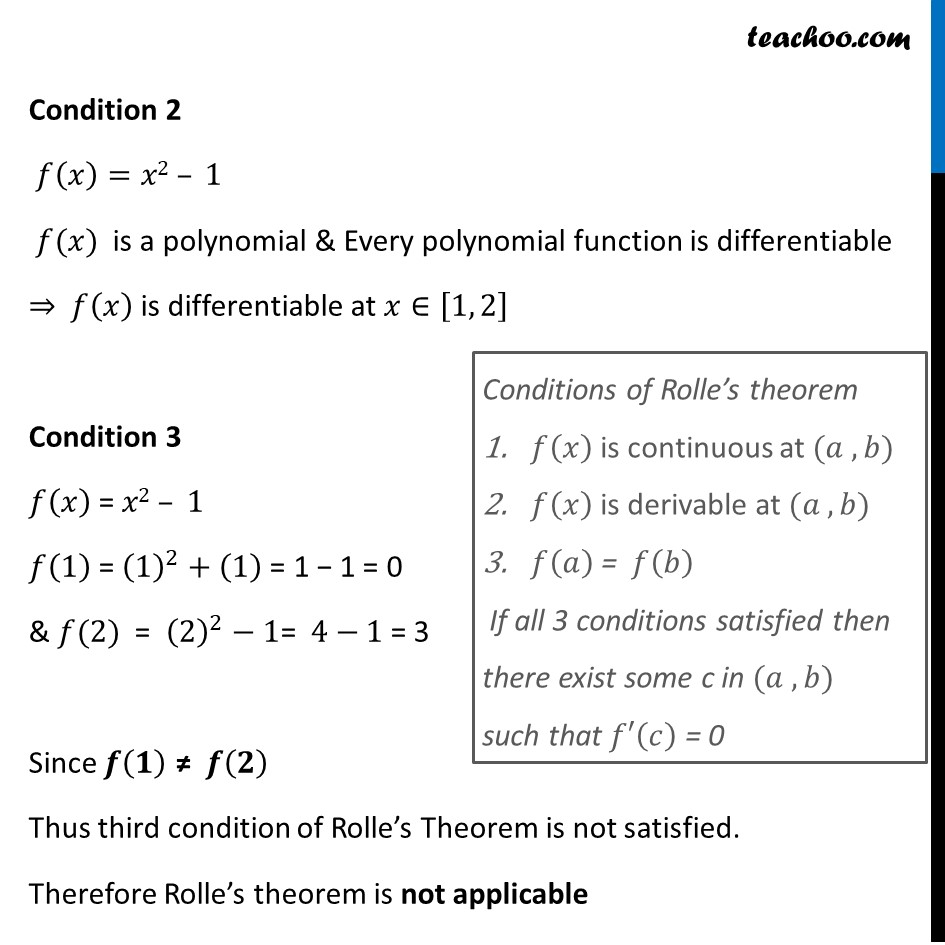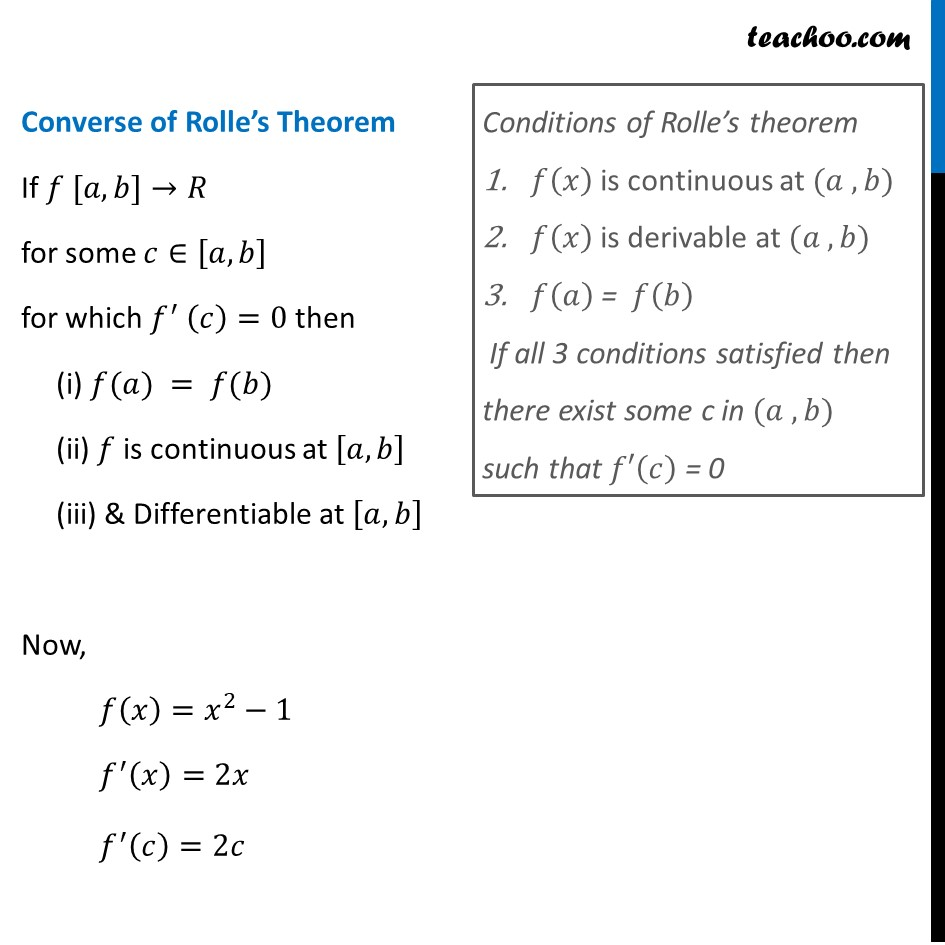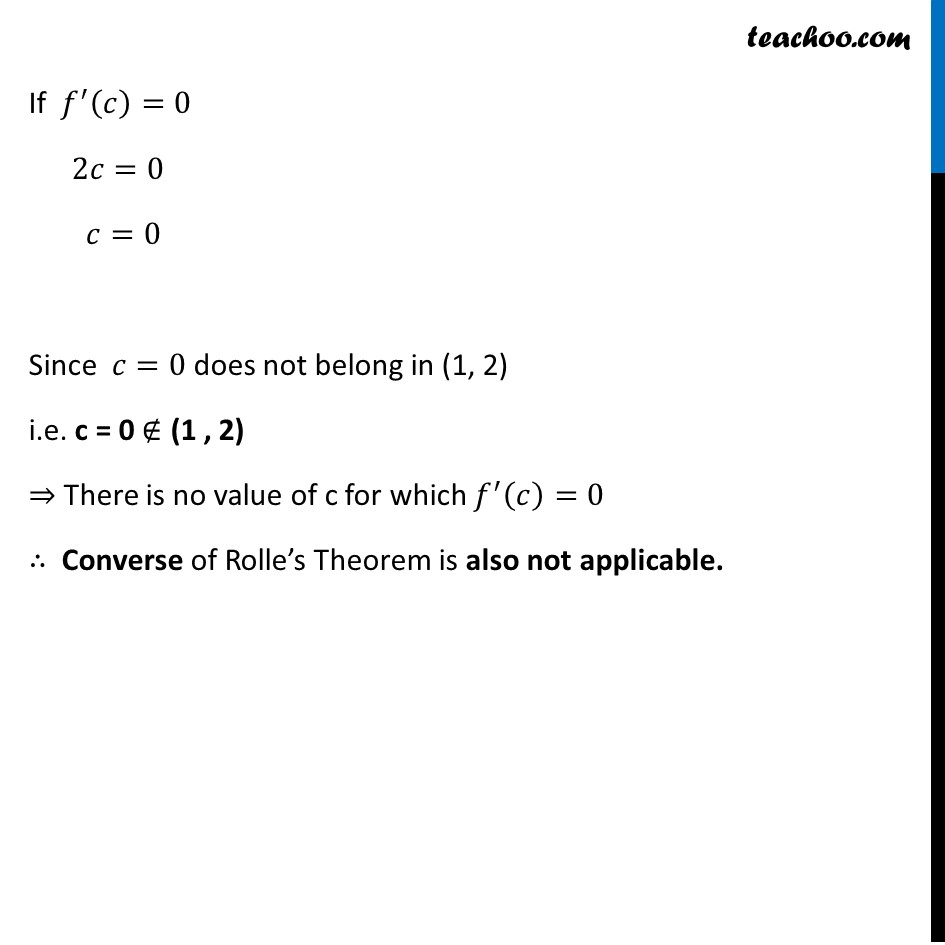Rolle's and Mean Value Theorem

Chapter 5 Class 12 Continuity and Differentiability
Serial order wiseLearn in your speed, with individual attention - Teachoo Maths 1-on-1 Class

### Transcript

Question 2 Examine if Rolle’s theorem is applicable to the functions. Can you say some thing about the converse of Rolle’s theorem from this function? (𝑖𝑖𝑖) 𝑓 (𝑥) = 𝑥2 – 1 𝑓𝑜𝑟 𝑥 ∈ [1, 2]𝑓 (𝑥) = 𝑥2 – 1 𝑓𝑜𝑟 𝑥 ∈ [1 , 2] Condition 1 𝑓(𝑥) = 𝑥2 – 1 𝑓(𝑥) is a polynomial & Every polynomial function is continuous ⇒ 𝑓(𝑥) is continuous at 𝑥∈[1, 2] Conditions of Rolle’s theorem 𝑓(𝑥) is continuous at (𝑎 , 𝑏) 𝑓(𝑥) is derivable at (𝑎 , 𝑏) 𝑓(𝑎) = 𝑓(𝑏) If all 3 conditions satisfied then there exist some c in (𝑎 , 𝑏) such that 𝑓′(𝑐) = 0 Condition 2 𝑓(𝑥)=𝑥2 – 1 𝑓(𝑥) is a polynomial & Every polynomial function is differentiable ⇒ 𝑓(𝑥) is differentiable at 𝑥∈[1, 2] Condition 3 𝑓(𝑥) = 𝑥2 – 1 𝑓(1) = (1)^2+(1) = 1 − 1 = 0 & 𝑓(2) = (2)^2−1= 4−1 = 3 Since 𝒇(𝟏) ≠ 𝒇(𝟐) Thus third condition of Rolle’s Theorem is not satisfied. Therefore Rolle’s theorem is not applicable Conditions of Rolle’s theorem 𝑓(𝑥) is continuous at (𝑎 , 𝑏) 𝑓(𝑥) is derivable at (𝑎 , 𝑏) 𝑓(𝑎) = 𝑓(𝑏) If all 3 conditions satisfied then there exist some c in (𝑎 , 𝑏) such that 𝑓′(𝑐) = 0 Converse of Rolle’s Theorem If 𝑓 [𝑎, 𝑏]→𝑅 for some 𝑐∈[𝑎, 𝑏] for which 𝑓^′ (𝑐)=0 then (i) 𝑓(𝑎) = 𝑓(𝑏) (ii) 𝑓 is continuous at [𝑎, 𝑏] (iii) & Differentiable at [𝑎, 𝑏] Now, 𝑓(𝑥)=𝑥^2−1 𝑓^′ (𝑥)=2𝑥 𝑓^′ (𝑐)=2𝑐 Conditions of Rolle’s theorem 𝑓(𝑥) is continuous at (𝑎 , 𝑏) 𝑓(𝑥) is derivable at (𝑎 , 𝑏) 𝑓(𝑎) = 𝑓(𝑏) If all 3 conditions satisfied then there exist some c in (𝑎 , 𝑏) such that 𝑓′(𝑐) = 0 If 𝑓^′ (𝑐)=0 2𝑐=0 𝑐=0 Since 𝑐=0 does not belong in (1, 2) i.e. c = 0 ∉ (1 , 2) ⇒ There is no value of c for which 𝑓^′ (𝑐)=0 ∴ Converse of Rolle’s Theorem is also not applicable.